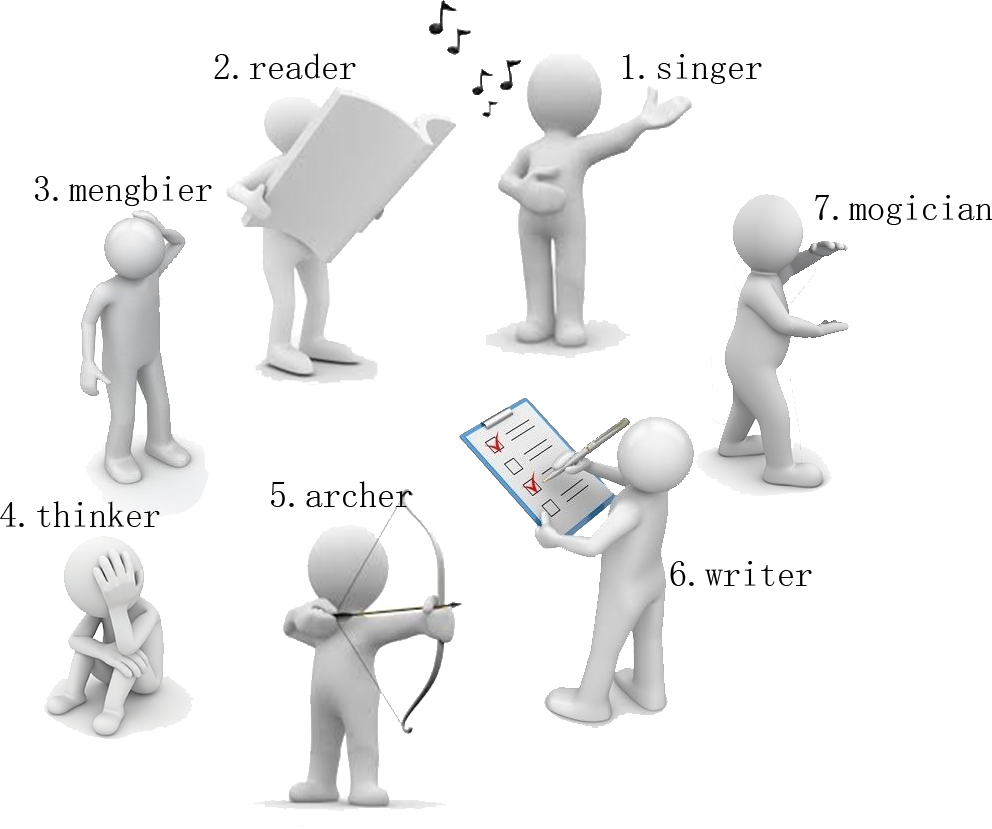# #260. 【NOIP2016】玩具谜题singer 朝内，左数第 $3$ 个是 archer

archer 朝外，右数第 $1$ 个是 thinker

thinker 朝外，左数第 $2$ 个是 writer

“所以眼镜藏在 writer 这里！”

### 样例一

#### input

7 3
0 singer
0 mengbier
1 thinker
1 archer
0 writer
1 mogician
0 3
1 1
0 2



#### output

writer



### 样例二

#### input

10 10
1 c
0 r
0 p
1 d
1 e
1 m
1 t
1 y
1 u
0 v
1 7
1 1
1 4
0 5
0 3
0 1
1 6
1 2
0 8
0 4



#### output

y



### 限制与约定

2×
3×
4×
5×
6×
7×
8×
9×
10×
11×
12×
13×
14×
15×
16×
17$=10^{5}$$=10^{5}$
18×
19×
20×

• 全朝内：若为“√”，表示该测试点保证所有的玩具小人都朝向圈内；
• 全左数：若为“√”，表示该测试点保证所有的指令都向左数，即对任意的 $1 \le i \le m$，$a_i = 0$；
• $s_i=1$：若为“√”，表示该测试点保证所有的指令都只数 $1$ 个，即对任意的 $1 \le i \le m$，$s_i = 1$；
• 职业长度为$1$：若为“√”，表示该测试点保证所有玩具小人的职业一定是一个长度为 $1$ 的字符串。